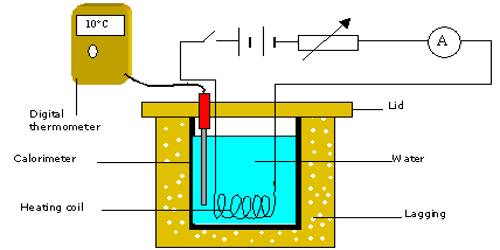# Joule’s laws for the Generation of Heat

Joule’s laws for the Generation of Heat

In 1841, the famous English scientist J. P. Joule from the experimental results stated three laws relating to heat generated due to the flow of current through a conductor. In honor of Joule, these laws are called Joule’s laws of heat. Those laws are stated below:

(1) Law of current: The amount of heat produced in a current conducting wire, is proportional to the square of the amount of current that is flowing through the wire when the electrical resistance of the wire and the time of current flowing are constant. If the resistance R of the conductor and time t of flow of current remain constant, the heat generated due to the flow of current ‘i’ is directly proportional to the square of the current.

i.e., H ∞ i2, if R and t remain constant.

Meaning of this law: If the current in the conductor becomes double, the heat generated will be four times the initial heat. If the current is made half, generated heat will be one-fourth of the initial value.

If currents i1, i2, i3, … … flow through a conductor for the same duration and respective heat produced are H1, H2, H3 …., then according to this law.

H1/ i1 = H2/ i2 = H3/ i3 = Constant

(2) Law of resistance: The amount of heat produced is proportional to the electrical resistance of the wire when the current in the wire and the time of current flowing are constant. If current and time of flow remain constant, then heat produced in a conductor due to the flow of current is directly proportional to the resistance of the conductor. i.e., H ∞ R, if ‘i’ and ‘t’ remain constant.

Meaning of this law: If the resistance of the conductor is either double or half, then heat produced in the conductor will be respectively double or half.

So, if current ‘i’ flows for time t through resistors R1, R2, R3, … connected in series and generated heats are respectively H1, H2, H3, …, then,

H1/ R1 = H2/ R2 = H3/ R3 = Constant

(3) Law of time: The heat generated due to the flow of current is proportional to the time of current flowingElecwhen the electrical resistance and the amount of current are constant. If the resistance of a conductor and current flowing through it remain constant, then the heat generated in the conductor due to the flow of current is directly proportional to the time of flow of current.

i.e., H ∞ t, if ‘i’ and ‘R’ are constant.

Meaning of this law: If the time of flow of current becomes either double or four generated heat in the conductor will be respectively double or four times the initial value.

So, for the same current flowing through a resistor for t1, t2, t3, … seconds and the generated heat in the conductor are respectively H1, H2, H3, …., then,

H1/ t1 = H2/ t2 = H3/ t3 = Constant

Combining the above three laws, we get,

H ∞ i2Rt = Ki2Rt …. ….. …. (1)

Here K is proportionality constant. It depends on the units of different quantities equation (1). If H is expressed in calorie, ‘i’ in ampere, R in ohm and t in sec., then, K = 0.24, i.e., K = 1/J.